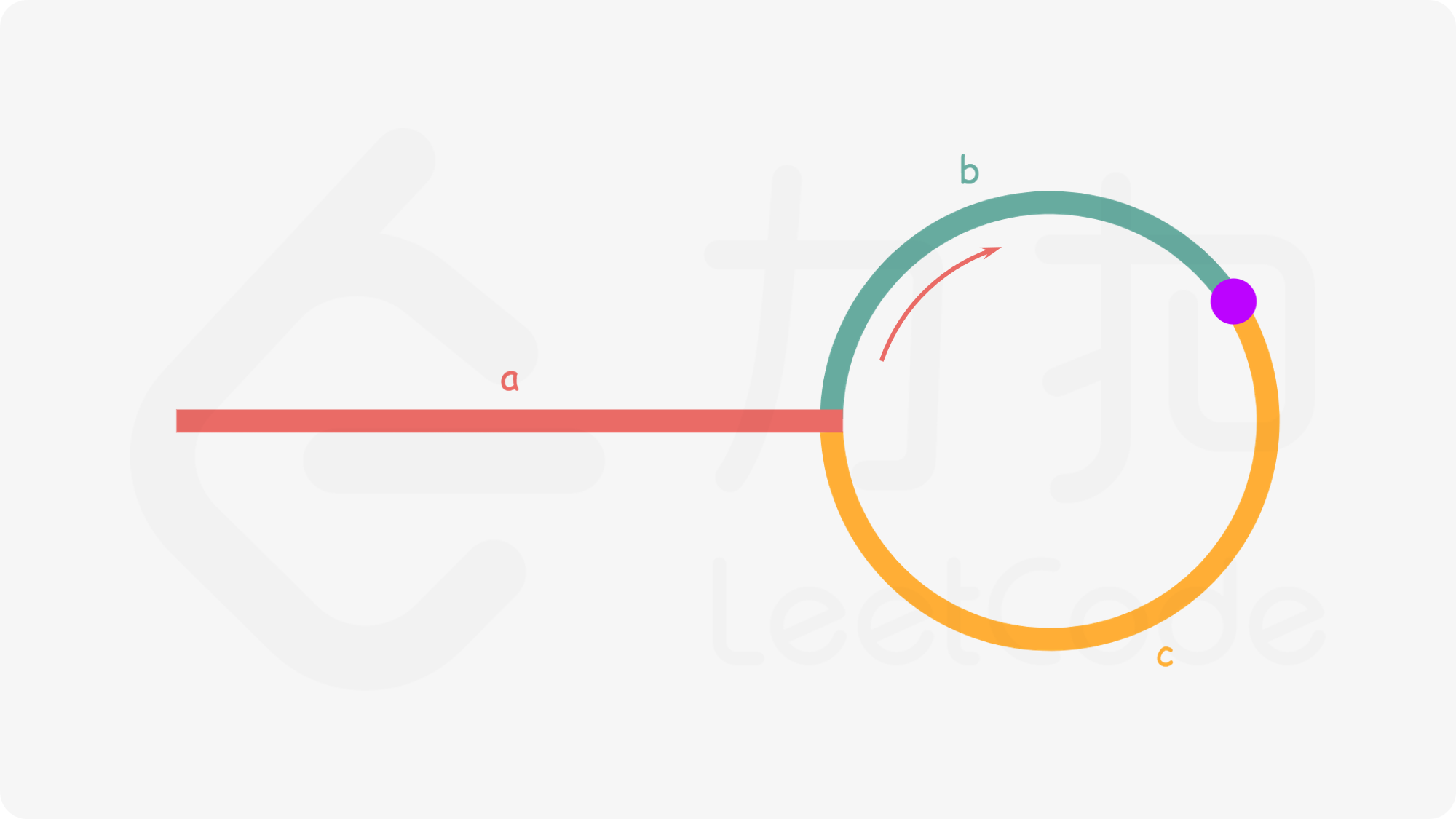# PHP 快慢指针的进阶题

## PHP 快慢指针的进阶题

author: he xiaodong date: 2020-10-10

##### 解题思路 2a + (n + 1)b + nc = 2(a+b) ⟹ a = c + (n − 1)(b + c)

``````/**
* Definition for a singly-linked list.
* class ListNode {
*     public \$val = 0;
*     public \$next = null;
*     function __construct(\$val) { \$this->val = \$val; }
* }
*/

class Solution {
/**
* @return ListNode
*/

// 在相遇点跳出循环

while (\$fast != null && \$fast->next != null) {
\$fast = \$fast->next->next;
\$slow = \$slow->next;
if (\$fast === \$slow) {
break;
}
}

// 声明 ptr 指针，步长为 1 的向前走

while (\$ptr !== \$slow) {
\$ptr = \$ptr->next;
\$slow = \$slow->next;
}
// ptr 即为入口

return \$ptr;
}
}
``````

##### 寻找重复数

``````输入: [1,3,4,2,2]

``````

``````输入: [3,1,3,4,2]

``````

##### 代码
``````class Solution {

/**
* @param Integer[] \$nums
* @return Integer
*/
function findDuplicate(\$nums) {
\$slow = 0;
\$fast = 0;
\$slow = \$nums[\$slow];
\$fast = \$nums[\$nums[\$fast]];
while(\$slow != \$fast){
\$slow = \$nums[\$slow];
\$fast = \$nums[\$nums[\$fast]];
}

\$pre1 = 0;
\$pre2 = \$slow;
while(\$pre1 != \$pre2){
\$pre1 = \$nums[\$pre1];
\$pre2 = \$nums[\$pre2];
}
return \$pre1;
}
}
``````